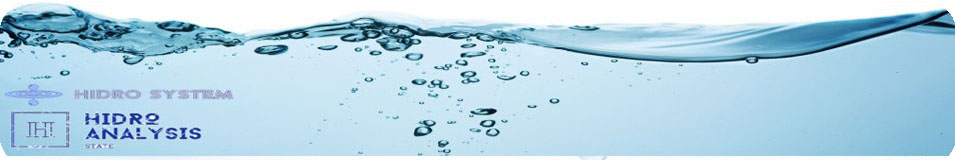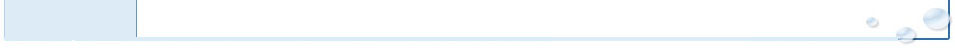# Formulas and Conversions

 Required capacity of Gas Chlorinator: GPM * 0.012 * PPM = PPD Required capacity of 12.5% Sodium Hypochlorite: GPM * 0.012 * PPM = GPD          "GPD/24 = GPH" Gas to 12.5% Sodium Hypochlorite Approximation: GPD = PPD

Where:

• GPM=Maximum quantity of water to be treated in gallons-per-minute
• PPM=Maximum desired chlorine concentration of the treated water in parts-per-million
• PPD=Minimum amount of chlorine required in pounds-per-day
• GPD=Minimum amount of 12.5% sodium hypochlorite required in gallons-per-day

Conversion Factors

1 lb = 0.453 kg
1 kg = 2.2 lb
1 oz = 30 g
1 g = 0.0335 oz
1 qt = 0.95 L
1 L = 1.06 qt
1 gal = 3.8L
1 L = 0.26 gal
°F = (9/5*°C) +32
°C = (°F -32)*(5/9)
1 ft = 30cm
1 cm = 0.04 ft
1 yd = 0.9m
1 m = 1.1 yd
1 PPM = 1 g/cm³ = 1 mg/L
1 GPM = 2.28*10-3 ft³/second